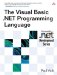# Precedence

 <  Day Day Up  >

All operators in Visual Basic .NET have a certain precedence that determines in what order operations are performed if an expression has more than one operator. An operator with higher precedence than another operator will be evaluated first. Operators with equal precedence will be evaluated from left to right. (Thus, operators are left-associative. )

For example, the multiplication operator ( * ) has a higher precedence than the addition operator ( + ). Therefore, the result of the expression 2 * 4 + 2 is 10 and not 12 . This is because the expression is evaluated as (2 * 4) + 2 , not 2 * (4 + 2) . The expression 2 * 4 / 2 is evaluated as (2 * 4) / 2 instead of 2 * (4 / 2) because the multiplication operator ( * ) has a precedence equal to the division operator ( / ).

## Style

It is preferable to use parentheses to clarify how an operation is to be performed rather than relying on the precedence and associativity of operators. It is easy to forget the precedence order of the operators!

Table 5-1 lists the operators in order of precedence. Each box represents a different level of precedence, from highest to lowest . All operators at the same level have the same precedence.

##### Table 5-1. Operator Precedence

Precedence

Description

Exponentiation

x ^ y

Unary plus

Unary minus

+ x

“ x

Multiplication

Division

x * y

x / y

Integer division

x \ y

Integer remainder

x Mod y

Subtraction

x + y

x “ y

Concatenation

x & y

Shift

x << y

x >> y

Equality

Inequality

Less than

Greater than

Less than or equal to

Greater than or equal to

Type equality

Reference equality

String matching

x = y

x <> y

x < y

x > y

x <= y

x >= y

TypeOf x Is y

x Is y

x Like y

Bitwise NOT

Not x

Bitwise AND

Logical AND

x And y

x AndAlso y

Bitwise OR

Logical OR

Bitwise XOR

x Or y

x OrElse y

x Xor y

 <  Day Day Up  >The Visual Basic .NET Programming Language
ISBN: 0321169514
EAN: 2147483647
Year: 2004
Pages: 173
Authors: Paul Vick

Similar book on Amazon